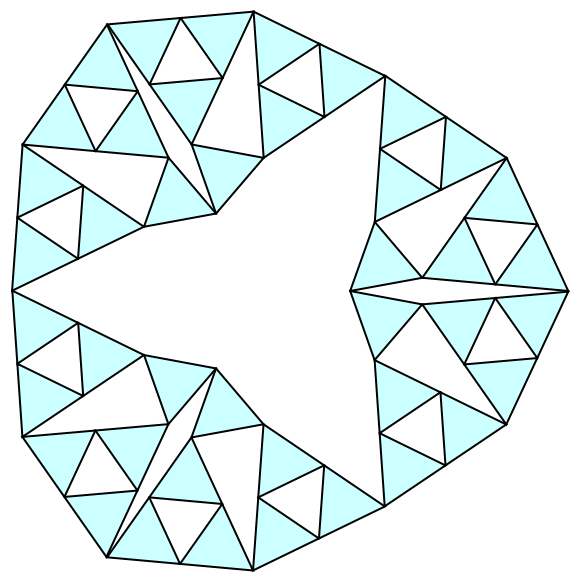Macalester Puzzles

The following puzzles were used as the puzzle of the week at the Macalester College Problem of the Week.

1. (week of November 5, 2002)

Find an arrangement of 50 non-overlapping unit squares so that each one touches exactly 4 others.

2. (week of October 15, 2017)

The positive integers x = 692 and y = 1118 have a curious property:

x2 + y2 = 1728788
x3 + y3 = 1728788920

The sum of their cubes starts with the digits of the sum of their squares. There are other pairs of positive integers with this property. Find another such pair.

3. (week of August 15, 2018)

Start with n players each with \$1. Each round, two players X and Y with money left are chosen randomly, and a coin is flipped. If it is heads, X pays Y \$1, and if it is tails, Y pays X \$1. What is the expected number of rounds until one person has all \$n?

4. (week of April 22, 2019)

Find a finite set of interior-disjoint unit squares in the plane such that each square touches exactly three others.

Find a finite set of interior-disjoint 2×1 dominoes in the plane such that each one touches exactly four others.

Here "touches" means that the intersection is a line segment that is more than a point.

5. (week of May 30, 2019)

You are the director of a tournament involving four players, A, B, C, D. The players are linearly ordered in terms of ability (perhaps C is better than A is better than B is better than D), but you do not know this order. Your job is to design a tournament that consists of a series of N games in order to maximize the probability that the best player is the champion. In every game between two players, the better player has a 2/3 chance of winning. The probability here is over the randomness in the game results and the randomness (as far as the director is concerned) in the ability-ordering of the players (all orderings are equally likely).

For example, if N = 3, the best tournament is A vs. B, C vs. D, and then the two winners against each other. The best player will win with probability (2/3)(2/3) or 44.4% of the time. The tournament with A vs. B, winner vs. C, and winner of previous vs. D is not as good. The best player wins that tournament with probability (2/4)(2/3)3 + (1/4)(2/3)2 + (1/4)(2/3) = 42.6%.

For N = 7, the best known tournament is A vs. B, C vs. D, and then a best-of-5 between the winners. Here the best player wins with probability (2/3) [(2/3)5 + 5 (2/3)4 (1/3) + 10 (2/3)3 (1/3)2] = 52.7%. An alternative is: A and B play a best-of-3, C plays D in a best-of-3, and the winners play each other; here the best player wins with probability [(2/3)3 + 3 (2/3)2 (1/3)](2/3) = 49.4%.

Suppose you have N=8 games at your disposal. Design a tournament that has the best player winning more than 53% of the time.

6. (week of November 19, 2020)

Call a set of equilateral triangles "saturated" if the triangles are interior disjoint, and whose edges are interior disjoint, yet every vertex is on exactly two of the triangles. Below is a set of 42 saturated congruent triangles.a) Find a set of 12 equilateral triangles of two different sizes that is saturated.

b) Find a set of 10 equilateral triangles of three different sizes that is saturated.

c) Find a set of 10 equilateral triangles with integer sides that is saturated.

7. (week of May 1, 2022)

Alice has 25 coins that look identical but have weights 1, 2, ...., 25 ounces. The coins are marked with labels 1 through 25 and Alice knows that all the labels are correct. Bob knows that the weights are 1 through 25, but knows nothing about the labels. How can she convince Bob that at least one of the labels is correct using just a single weighing on a balance scale?

8. (week of March 23, 2023)

Suppose there are 8 teams in a single elimination tournament, seeded in the usual way from #1 (best) to #8 (worst). In the first round, #1 plays #8, #4 plays #5 (and the winner of 1 vs 8 then plays the winner of 4 vs 5), and #2 plays #7, #3 plays #6 (and the winner of 3 vs 6 then plays the winner of 2 vs 7). The two unbeaten teams after the second round play in the final game to determine the tournament winner.

Suppose the lower seed (the better team) wins with fixed probability p, where 1/2 ≤ p ≤ 1. The #1 seed would prefer p=1 as that guarantees that they win every game. What value of p would team #2 want so as to maximize its chance of winning the tournament? Same question for the other six teams.